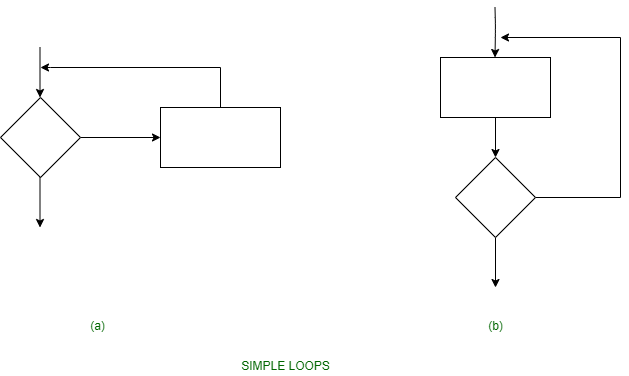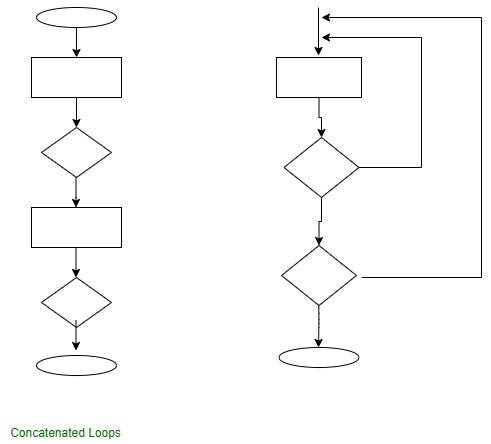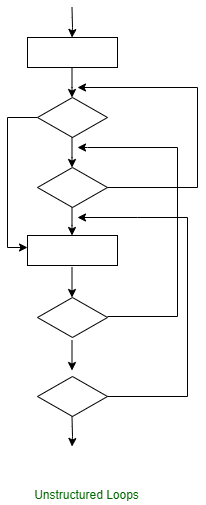# Control Structure Testing

• Last Updated : 07 Apr, 2020

Control structure testing is used to increase the coverage area by testing various control structures present in the program. The different types of testing performed under control structure testing are as follows-

```1. Condition Testing
2. Data Flow Testing
3. Loop Testing ```

1. Condition Testing :
Condition testing is a test cased design method, which ensures that the logical condition and decision statements are free from errors. The errors present in logical conditions can be incorrect boolean operators, missing parenthesis in a booleans expression, error in relational operators, arithmetic expressions, and so on.

Attention reader! Don’t stop learning now. Get hold of all the important CS Theory concepts for SDE interviews with the CS Theory Course at a student-friendly price and become industry ready.

The common types of logical conditions that are tested using condition testing are-

1. A relation expression, like E1 op E2 where ‘E1’ and ‘E2’ are arithmetic expressions and ‘OP’ is an operator.
2. A simple condition like any relational expression preceded by a NOT (~) operator.
For example, (~E1) where ‘E1’ is an arithmetic expression and ‘a’ denotes NOT operator.
3. A compound condition consists of two or more simple conditions, Boolean operator, and parenthesis.
For example, (E1 & E2)|(E2 & E3) where E1, E2, E3 denote arithmetic expression and ‘&’ and ‘|’ denote AND or OR operators.
4. A Boolean expression consists of operands and a Boolean operator like ‘AND’, OR, NOT.
For example, ‘A|B’ is a Boolean expression where ‘A’ and ‘B’ denote operands and | denotes OR operator.

2. Data Flow Testing :
The data flow test method chooses the test path of a program based on the locations of the definitions and uses all the variables in the program.

The data flow test approach is depicted as follows suppose each statement in a program is assigned a unique statement number and that theme function cannot modify its parameters or global variables.
For example, with S as its statement number.

```DEF (S) = {X | Statement S has a definition of X}
USE (S) = {X | Statement S has a use of X} ```

If statement S is an if loop statement, them its DEF set is empty and its USE set depends on the state of statement S. The definition of the variable X at statement S is called the line of statement S’ if the statement is any way from S to statement S’ then there is no other definition of X.

A definition use (DU) chain of variable X has the form [X, S, S’], where S and S’ denote statement numbers, X is in DEF(S) and USE(S’), and the definition of X in statement S is line at statement S’.

A simple data flow test approach requires that each DU chain be covered at least once. This approach is known as the DU test approach. The DU testing does not ensure coverage of all branches of a program.

However, a branch is not guaranteed to be covered by DU testing only in rar cases such as then in which the other construct does not have any certainty of any variable in its later part and the other part is not present. Data flow testing strategies are appropriate for choosing test paths of a program containing nested if and loop statements.

3. Loop Testing :
Loop testing is actually a white box testing technique. It specifically focuses on the validity of loop construction.
Following are the types of loops.

1. Simple Loop – The following set of test can be applied to simple loops, where the maximum allowable number through the loop is n.
1. Skip the entire loop.
2. Traverse the loop only once.
3. Traverse the loop two times.
4. Make p passes through the loop where p<n.
5. Traverse the loop n-1, n, n+1 times.2. Concatenated Loops – If loops are not dependent on each other, contact loops can be tested using the approach used in simple loops. if the loops are interdependent, the steps are followed in nested loops.3. Nested Loops – Loops within loops are called as nested loops. when testing nested loops, the number of tested increases as level nesting increases.
The following steps for testing nested loops are as follows-
1. Start with inner loop. set all other loops to minimum values.
2. Conduct simple loop testing on inner loop.
3. Work outwards.
4. Continue until all loops tested.
4. Unstructured loops – This type of loops should be redesigned, whenever possible, to reflect the use of unstructured the structured programming constructs.My Personal Notes arrow_drop_up